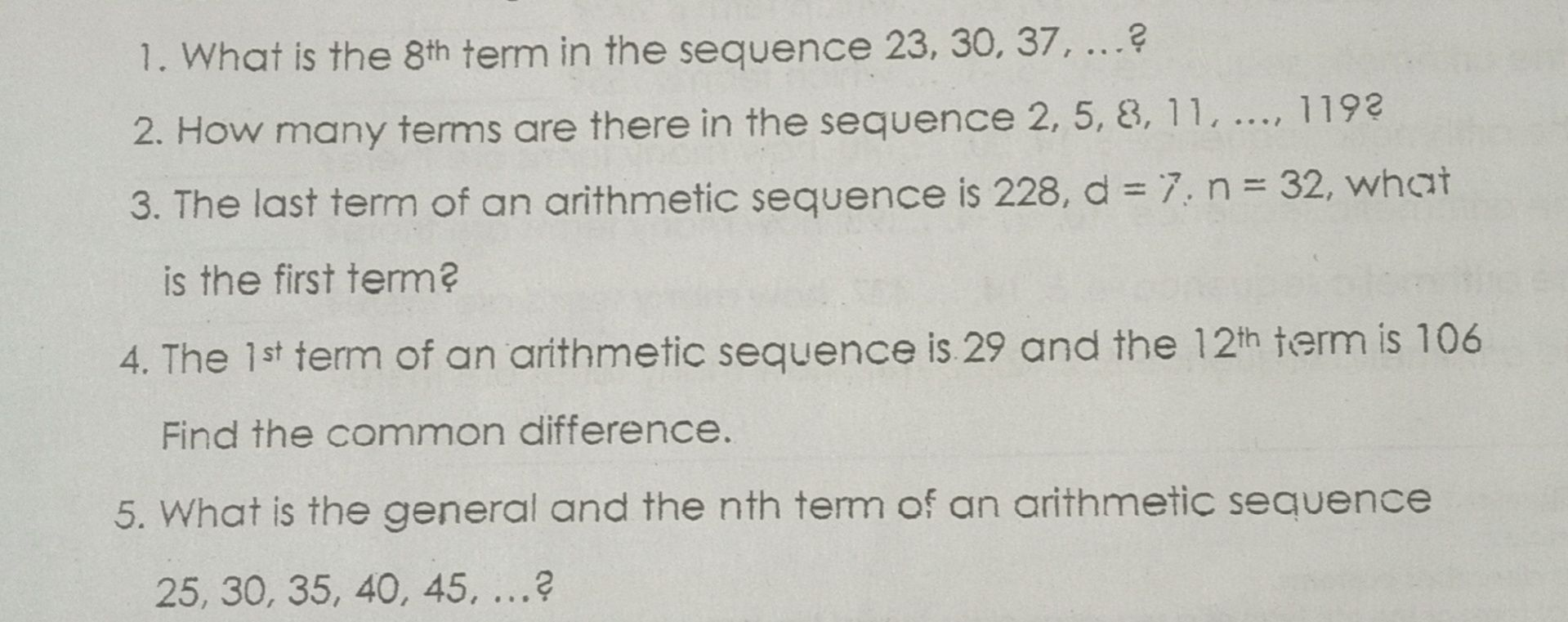### ¿Todavía tienes preguntas de matemáticas?

Pregunte a nuestros tutores expertos
Algebra
Pregunta1. What is the $$8 ^ { th}$$ term in the sequence $$23,30,37 , \ldots$$ ?

2. How many terms are there in the sequence $$2,5 , 8 , 11 , \ldots , 119 ?$$

3. The last term of an arithmetic sequence is $$228 , d = 7 . n = 32$$ , what is the first term?

4. The $$1 ^ { st}$$ term of an arithmetic sequence is $$29$$ and the $$12 ^ { th}$$ term is $$106$$ Find the common difference.

5. What is the general and the nth term of an arithmetic sequence $$25,30,35,40,45 , \ldots$$ ?

An=A1-(n-1)d

1. 72

2. 40

3. 11

4. 7

5. An=5n+20

Solución
View full explanation on CameraMath App.